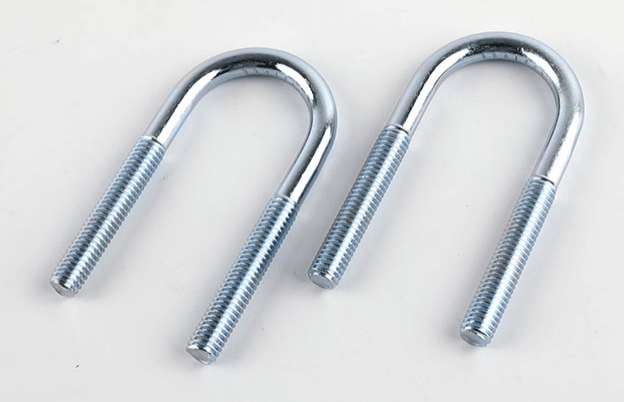# Bolt tensile strength and yield strength

Updated:2021-03-05
Summary:Bolt tensile strength and yield strength. So what ...

Bolt tensile strength and yield strength. So what is the tensile strength and yield strength? Today I will talk with you.

In general, under certain external force, the material will be damaged beyond a certain limit. In general, there are many forces that cause damage to materials, such as tensile force, pressure, shear force, and torque.

The tensile strength and yield strength that we talk about today are all based on tensile strength and are obtained through tensile testing. Generally, the material is stretched through a tensile machine until it breaks or reaches a certain degree of damage. And the force that causes ultimate damage to the material is the tensile ultimate load of the material. Newton N or kilonewton KN is generally used to express the pulling force. Divide the ultimate tensile force by the cross-sectional area of ​​the experimental material to obtain the ultimate tensile load per unit area. The force per unit area is generally expressed in megapascals (MPa = N / mm).Tensile strength is the ratio of the tensile ultimate load divided by the cross-sectional area of ​​the material. The tensile strength is the limit of the maximum external force that the material can withstand per unit area. Above this limit, the material will be destroyed.

What is the yield strength? Yield strength is for elastic materials. Inelastic materials have no yield strength. For example, all kinds of metal materials, plastics, rubber, etc. have yield strength. However, glass, ceramics, masonry, etc. are relatively brittle, and generally have no elasticity or yield strength.

Generally, the material undergoes elastic deformation under the action of external force (what is elastic deformation? It means that the external force is eliminated and the material will restore its original size and shape). When the external force continues to increase, the material will enter plastic deformation after a certain value is reached. Once the material enters plastic deformation and the external force is eliminated, the original size and shape of the material cannot be restored! And the critical point strength that causes two kinds of deformation is the yield strength of the material! Corresponding to the applied tension, the value of this critical point is called the yield point.